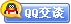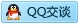##用户名 Email 自动登录 找回密码 密码 会员注册
 VIP会员，3年作业免费下 ！ 奥鹏作业，奥鹏毕业论文检测 新手作业下载教程，充值问题 没有找到答案，请在此处留言！ 2020年07月最新全国统考资料 投诉建议，加盟合作！奥鹏课程积分软件(ver:3.1)

# 12秋浙大《微积分(1)》在线作业发表于 2012-9-21 11:33:18 | 显示全部楼层 |阅读模式一、单选题（共 25 道试题，共 50 分。）V 1.  设函数f(x)在[-a, a](a>0)上是偶函数，则 |f(-x)| 在[-a, a]上是() A. 奇函数 B. 偶函数 C. 非奇非偶函数 D. 可能是奇函数，也可能是偶函数       满分：2  分 2.  试题如图 A. B. C. D.       满分：2  分 3.  对于函数f(x)=[(x^2-1)(x^2-4)]^(2/3),下列能满足罗尔定理条件的区间是 A. [0,√5] B. [-1,1] C. [-2,1] D. [-1,2]       满分：2  分 4.  当x趋于1时，1/Inx-1/(x-1)的极限为() A. -1/2 B. 1/2 C. 0 D. 1       满分：2  分 5.  试题如图 A. B. C. D.       满分：2  分 6.  g(x)=1+x,x不等0时，f[g（x）]=(2-x)/x,则f‘(0)=() A. 2 B. -2 C. 1 D. -1       满分：2  分 7.  y=logax（a>0，a≠1为常数）的导数为() A. 1/(xIna) B. Ina/x C. x/Ina D. xIna       满分：2  分 8.  曲线y=x^2+x-2在点(1.5,1.75)处的切线方程为() A. 16x-4y-17=0 B. 16x+4y-31=0 C. 2x-8y+11=0 D. 2x+8y-17=0       满分：2  分 9.  x=0是函数f(x)=x arctan(1/x)的 A. 连续点 B. 可去间断点 C. 跳跃间断点 D. 无穷间断点       满分：2  分 10.  设f(-1)=0,f(0)=2,f(1)=-3,f(2)=5,三次有理函数f(x)=ax3+bx2+cx+d中abcd的值分别为() A. a=10/3,b=-7/2,c=-29/6,d=2 B. a=-10/3,b=7/2,c=-29/6,d=2 C. a=10/3,b=7/2,c=-29/6,d=-2 D. a=-10/3,b=-7/2,c=-29/6,d=-2       满分：2  分 11.  下列集合中为空集的是 A. {x|e^x=1} B. {0} C. {(x, y)|x^2+y^2=0} D. {x| x^2+1=0,x∈R}       满分：2  分 12.  试题如图 A. B. C. D.       满分：2  分 13.  若x->x0,lim f(x)=A,则必有（） A. f(x)在x0的某个去心领域有界 B. f(x)在x0的任意去心领域有界 C. f(x)在x0的某个去心领域无界 D. f(x)在x0的任意去心领域无界       满分：2  分 14.  试题如图 A. B. C. D.       满分：2  分 15.  当x趋于0时，cotx-1/x的极限为() A. 0 B. 1 C. -1 D. 2       满分：2  分 16.  f(x)严格单调增加函数，则下列函数不一定严格单调递增的是（） A. [f(x)]^2 B. f[f(x)] C. e^f(x) D. f(e^x)       满分：2  分 17.  若函数f(x,y)在(x0,y0)的某邻域内连续，则函数f(x0,y)() A. 在y0点连续 B. 在y0点可导 C. 在y0点可微 D. 在y0点取得极值       满分：2  分 18.  试题如图 A. B. C. D.       满分：2  分 19.  试题如图 A. B. C. D.       满分：2  分 20.  函数y=c(c为常数)的导函数为() A. y'=0 B. y'=1 C. y'=1/c D. y'=c       满分：2  分 21.  设f(x)=x(x-1)(x-2)(x-3),则f’(0)=() A. -6 B. -2 C. 3 D. -3       满分：2  分 22.  试题如图 A. B. C. D.       满分：2  分 23.  试题如图 A. B. C. D.       满分：2  分 24.  试题如图 A. B. C. D.       满分：2  分 25.  试题如图 A. B. C. D.       满分：2  分 二、判断题（共 25 道试题，共 50 分。）V 1.  有限多个无穷小量之和仍是无穷小。 A. 错误 B. 正确       满分：2  分 2.  第一类间断点的特点是左右极限都不存在。 A. 错误 B. 正确       满分：2  分 3.  两个无穷大量之和仍是无穷大。 A. 错误 B. 正确       满分：2  分 4.  函数可导的充要条件是左右导数都存在且相等。 A. 错误 B. 正确       满分：2  分 5.  一元函数可导必可微，可微必可导。 A. 错误 B. 正确       满分：2  分 6.  图像法表示函数的特点是形象直观，一目了然。 A. 错误 B. 正确       满分：2  分 7.  数列收敛的充分必要条件是它的任一子数列都收敛且极限相等。 A. 错误 B. 正确       满分：2  分 8.  驻点或者导数不存在的点一定是函数单调区间的分界点。 A. 错误 B. 正确       满分：2  分 9.  函数反函数的导数等于其直接函数的导数之倒数。 A. 错误 B. 正确       满分：2  分 10.  若函数在闭区间上连续，则它不一定有界。 A. 错误 B. 正确       满分：2  分 11.  反函数的定义域是原函数的值域。 A. 错误 B. 正确       满分：2  分 12.  函数可用表格法，图像法或公式法表示。 A. 错误 B. 正确       满分：2  分 13.  无穷小量是一种很小的量。 A. 错误 B. 正确       满分：2  分 14.  y=x,y=sin(arcsinx)是同一个函数。 A. 错误 B. 正确       满分：2  分 15.  任何初等函数都是在它定义区间上的连续函数。 A. 错误 B. 正确       满分：2  分 16.  复合函数对自变量的导数等于函数对中间变量的导数乘以中间变量对自变量的导数。 A. 错误 B. 正确       满分：2  分 17.  分段函数是由几个函数组成的。 A. 错误 B. 正确       满分：2  分 18.  可去间断点属于第二类间断点。 A. 错误 B. 正确       满分：2  分 19.  若数列收敛，则数列的极限惟一。 A. 错误 B. 正确       满分：2  分 20.  若函数在某一点的极限存在，则它在这点的极限惟一。 A. 错误 B. 正确       满分：2  分 21.  函数的本质是指因变量y。 A. 错误 B. 正确       满分：2  分 22.  极值点一定包含在区间内部驻点或导数不存在的点之中。 A. 错误 B. 正确       满分：2  分 23.  设函数y=lnsecx，则y” =secx A. 错误 B. 正确       满分：2  分 24.  严格递增的函数必有严格递增的反函数。 A. 错误 B. 正确       满分：2  分 25.  函数图像在某点的余弦就是导数的几何意义。 A. 错误 B. 正确       满分：2  分

### 本帖子中包含更多资源

x
 本版积分规则 回帖并转播 回帖后跳转到最后一页客服一客服二客服三客服四微信客服扫一扫